Tamil Nadu Board of Secondary EducationSSLC (English Medium) Class 7th

# Tamil Nadu Board Samacheer Kalvi solutions for Class 7th Mathematics Term 1 Answers Guide chapter 2 - Measurements [Latest edition]

#### Chapters## Chapter 2: Measurements

Exercise 2.1Exercise 2.2Exercise 2.3Exercise 2.4
Exercise 2.1 [Pages 38 - 39]

### Tamil Nadu Board Samacheer Kalvi solutions for Class 7th Mathematics Term 1 Answers Guide Chapter 2 Measurements Exercise 2.1 [Pages 38 - 39]

Exercise 2.1 | Q 1. (i) | Page 38

Find the area and perimeter of the following parallelograms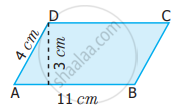Exercise 2.1 | Q 1. (ii) | Page 38

Find the area and perimeter of the following parallelograms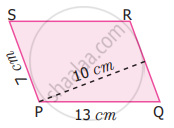Exercise 2.1 | Q 2. (i) | Page 39

Find the missing values.

 Base Height Area 18 cm 5 cm
Exercise 2.1 | Q 2. (ii) | Page 39

Find the missing values.

 Base Height Area 8 cm 56 sq.m
Exercise 2.1 | Q 2. (iii) | Page 39

Find the missing values.

 Base Height Area 17 mm 221 sq.mm
Exercise 2.1 | Q 3 | Page 39

Suresh on a parallelogram-shaped trophy in a state level chess tournament. He knows that the area of the trophy is 735 sq.cm and its base is 21 cm. What is the height of that trophy?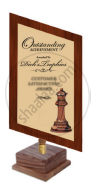Exercise 2.1 | Q 4 | Page 39

Janaki has a piece of fabric in the shape of a parallelogram. Its height is 12 m and its base is 18 m. She cuts the fabric into four equal parallelograms by cutting the parallel sides through its mid-points. Find the area of each new parallelogram

Exercise 2.1 | Q 5 | Page 39

A ground is in the shape of parallelogram. The height of the parallelogram is 14 metres and the corresponding base is 8 metres longer than its height. Find the cost of levelling the ground at the rate of ₹ 15 per sq.m

#### Objective type questions

Exercise 2.1 | Q 6 | Page 39

The perimeter of a parallelogram whose adjacent sides are 6 cm and 5 cm is

• 12 cm

• 10 cm

• 24 cm

• 22 cm

Exercise 2.1 | Q 7 | Page 39

The area of parallelogram whose base 10 m and height 7 m is

• 70 sq.m

• 35 sq.m

• 7 sq.m

• 10 sq.m

Exercise 2.1 | Q 8 | Page 39

The base of the parallelogram with area is 52 sq.cm and height 4 cm is

• 48 cm

• 104 cm

• 13 cm

• 26 cm

Exercise 2.1 | Q 9 | Page 39

What happens to the area of the parallelogram if the base is increased 2 times and the height is halved?

• Decreases to half

• Remains the same

• Increase by two times

• None

Exercise 2.1 | Q 10 | Page 39

In a parallelogram the base is three times its height. If the height is 8 cm then the area is

• 64 sq.cm

• 192 sq.cm

• 32 sq.cm

• 72 sq.cm

Exercise 2.2 [Page 43]

### Tamil Nadu Board Samacheer Kalvi solutions for Class 7th Mathematics Term 1 Answers Guide Chapter 2 Measurements Exercise 2.2 [Page 43]

Exercise 2.2 | Q 1. (i) | Page 43

Find the area of rhombus PQRS shown in the following figure.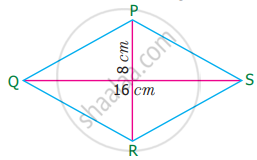Exercise 2.2 | Q 1. (ii) | Page 43

Find the area of rhombus PQRS shown in the following figure.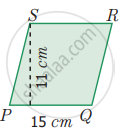Exercise 2.2 | Q 2 | Page 43

Find the area of a rhombus whose base is 14 cm and height is 9 cm.

Exercise 2.2 | Q 3. (i) | Page 43

Find the missing value.

 Diagonal (d1) Diagonal (d2) Area 19 cm 16 cm
Exercise 2.2 | Q 3. (ii) | Page 43

Find the missing value.

 Diagonal (d1) Diagonal (d2) Area 26 m 468 sq.m
Exercise 2.2 | Q 3. (iii) | Page 43

Find the missing value.

 Diagonal (d1) Diagonal (d2) Area 12 mm 180 sq.mm
Exercise 2.2 | Q 4 | Page 43

The area of a rhombus is 100 sq.cm and length of one of its diagonals is 8 cm. Find the length of the other diagonal

Exercise 2.2 | Q 5 | Page 43

A sweet is in the shape of rhombus whose diagonals are given as 4 cm and 5 cm. The surface of the sweet should be covered by an aluminum foil. Find the cost of aluminum foil used for 400 such sweets at the rate of ₹ 7 per 100 sq.cm

#### Objective type questions

Exercise 2.2 | Q 6 | Page 43

The area of the rhombus with side 4 cm and height 3 cm is

• 7 sq.cm

• 24 sq.cm

• 12 sq.cm

• 10 sq.cm

Exercise 2.2 | Q 7 | Page 43

The area of the rhombus when both diagonals measuring 8 cm is

• 64 sq.cm

• 32 sq.cm

• 30 sq.cm

• 16 sq.cm

Exercise 2.2 | Q 8 | Page 43

The area of the rhombus is 128 sq.cm and the length of one diagonal is 32 cm. The length of the other diagonal is

• 12 cm

• 8 cm

• 4 cm

• 20 cm

Exercise 2.2 | Q 9 | Page 43

The height of the rhombus whose area 96 sq.m and side 24 m is

• 8 m

• 10 m

• 2 m

• 4 m

Exercise 2.2 | Q 10 | Page 43

The angle between the diagonals of a rhombus is

• 120°

• 180°

• 90°

• 100°

Exercise 2.3 [Page 47]

### Tamil Nadu Board Samacheer Kalvi solutions for Class 7th Mathematics Term 1 Answers Guide Chapter 2 Measurements Exercise 2.3 [Page 47]

Exercise 2.3 | Q 1. (i) | Page 47

Find the missing values.

 Height 'h' Parallel side 'a Parallel side 'b Area 10 m 12 m 20 m
Exercise 2.3 | Q 1. (ii) | Page 47

Find the missing values.

 Height 'h' Parallel side 'a Parallel side 'b Area 13 cm 28 cm 492 sq.cm
Exercise 2.3 | Q 1. (iii) | Page 47

Find the missing values.

 Height 'h' Parallel side 'a Parallel side 'b Area 19 m 16 m 323 sq.m
Exercise 2.3 | Q 1. (iv) | Page 47

Find the missing values.

 Height 'h' Parallel side 'a Parallel side 'b Area 16 cm 15 cm 360 sq.cm
Exercise 2.3 | Q 2 | Page 47

Find the area of a trapezium whose parallel sides are 24 cm and 20 cm and the distance between them is 15 cm

Exercise 2.3 | Q 3 | Page 47

The area of a trapezium is 1586 sq.cm. The distance between its parallel sides is 26 cm. If one of the parallel sides is 84 cm then find the other side

Exercise 2.3 | Q 4 | Page 47

The area of a trapezium is 1080 sq.cm. If the lengths of its parallel sides are 55.6 cm and 34.4 cm. Find the distance between them

Exercise 2.3 | Q 5 | Page 47

The area of a trapezium is 180 sq.cm and its height is 9 cm. If one of the parallel sides is longer than the other by 6 cm. Find the length of the parallel sides.

Exercise 2.3 | Q 6 | Page 47

The sunshade of a window is in the form of isosceles trapezium whose parallel sides are 81 cm and 64 cm and the distance between them is 6 cm. Find the cost of painting the surface at the rate of ₹ 2 per sq.cm

Exercise 2.3 | Q 7 | Page 47

A window is in the form of trapezium whose parallel sides are 105 cm and 50 cm respectively and the distance between the parallel sides is 60 cm. Find the cost of the glass used to cover the window at the rate of ₹ 15 per 100 sq.cm

#### Objective type questions

Exercise 2.3 | Q 8 | Page 47

The area of the trapezium, if the parallel sides are measuring 8 cm and 10 cm and the height 5 cm is

• 45 sq.cm

• 40 sq.cm

• 18 sq.cm

• 50 sq.cm

Exercise 2.3 | Q 9 | Page 47

In a trapezium if the sum of the parallel sides is 10 cm and the area is 140 sq.cm, then the height is

• 7 cm

• 40 cm

• 14 cm

• 28 cm

Exercise 2.3 | Q 10 | Page 47

When the non-parallel sides of a trapezium are equal then it is known as

• a square

• a rectangle

• an isosceles trapezium

• a parallelogram

Exercise 2.4 [Pages 48 - 49]

### Tamil Nadu Board Samacheer Kalvi solutions for Class 7th Mathematics Term 1 Answers Guide Chapter 2 Measurements Exercise 2.4 [Pages 48 - 49]

#### Miscellaneous Practice problems

Exercise 2.4 | Q 1 | Page 48

The base of the parallelogram is 16 cm and the height is 7 cm less than its base. Find the area of the parallelogram

Exercise 2.4 | Q 2 | Page 48

An agricultural field is in the form of a parallelogram, whose area is 68.75 sq.hm. The distance between the parallel sides is 6.25 cm. Find the length of the base

Exercise 2.4 | Q 3 | Page 48

A square and a parallelogram have the same area. If the side of the square is 48 m and the height of the parallelogram is 18 m. Find the length of the base of the parallelogram

Exercise 2.4 | Q 4 | Page 48

The height of the parallelogram is one-fourth of its base. If the area of the parallelogram is 676 sq.cm, find the height and the base

Exercise 2.4 | Q 5 | Page 48

The area of the rhombus is 576 sq.cm and the length of one of its diagonal is half of the length of the other diagonal then find the length of the diagonal

Exercise 2.4 | Q 6 | Page 48

A ground is in the form of isosceles trapezium with parallel sides measuring 42 m and 36 m long. The distance between the parallel sides is 30 m. Find the cost of levelling it at the rate of ₹ 135 per sq.m

#### Challenge Problems

Exercise 2.4 | Q 7 | Page 48

In a parallelogram PQRS (See the diagram) PM and PN are the heights corresponding to the sides QR and RS respectively. If the area of the parallelogram is 900 sq.cm and the length of PM and PN are 20 cm and 36 cm respectively, find the length of the sides QR and SR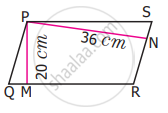Exercise 2.4 | Q 8 | Page 48

If the base and height of a parallelogram are in the ratio 7 : 3 and the height is 45 cm, then fixed the area of the parallelogram

Exercise 2.4 | Q 9 | Page 48

Find the area of the parallelogram ABCD if AC is 24 cm and BE = DF = 8 cm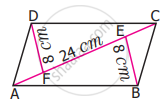Exercise 2.4 | Q 10 | Page 48

The area of the parallelogram ABCD is 1470 sq.cm. If AB = 49 cm and AD = 35 cm then, find the height, DF and BE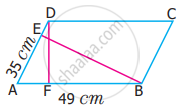Exercise 2.4 | Q 11 | Page 49

One of the diagonals of a rhombus is thrice as the other. If the sum of the length of the diagonals is 24 cm, then find the area of the rhombus.

Exercise 2.4 | Q 12 | Page 49

A man has to build a rhombus shaped swimming pool. One of the diagonal is 13 m and the other is twice the first one. Then find the area of the swimming pool and also find the cost of cementing the floor at the rate of ₹ 15 per sq.cm

Exercise 2.4 | Q 13 | Page 49

Find the height of the parallelogram whose base is four times the height and whose area is 576 sq.cm

Exercise 2.4 | Q 14 | Page 49

The table top is in the shape of a trapezium with measurements given in the figure. Find the cost of the glass used to cover the table at the rate of ₹ 6 per 10 sq.cm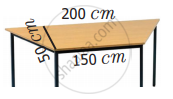Exercise 2.4 | Q 15 | Page 49

Arivu has a land ABCD with the measurements given in the figure. If a portion ABED is used for cultivation (where E is the mid-point of DC), find the cultivated area.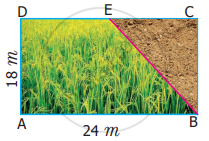## Chapter 2: Measurements

Exercise 2.1Exercise 2.2Exercise 2.3Exercise 2.4## Tamil Nadu Board Samacheer Kalvi solutions for Class 7th Mathematics Term 1 Answers Guide chapter 2 - Measurements

Tamil Nadu Board Samacheer Kalvi solutions for Class 7th Mathematics Term 1 Answers Guide chapter 2 (Measurements) include all questions with solution and detail explanation. This will clear students doubts about any question and improve application skills while preparing for board exams. The detailed, step-by-step solutions will help you understand the concepts better and clear your confusions, if any. Shaalaa.com has the Tamil Nadu Board of Secondary Education Class 7th Mathematics Term 1 Answers Guide solutions in a manner that help students grasp basic concepts better and faster.

Further, we at Shaalaa.com provide such solutions so that students can prepare for written exams. Tamil Nadu Board Samacheer Kalvi textbook solutions can be a core help for self-study and acts as a perfect self-help guidance for students.

Concepts covered in Class 7th Mathematics Term 1 Answers Guide chapter 2 Measurements are Concept of Perimeter, Concept of Area, Properties of a Parallelogram, Properties of Rhombus, Properties of Trapezium, Area of a Parallelogram, Perimeter of Parallelogram, Area of a Rhombus, Area of Trapezium.

Using Tamil Nadu Board Samacheer Kalvi Class 7th solutions Measurements exercise by students are an easy way to prepare for the exams, as they involve solutions arranged chapter-wise also page wise. The questions involved in Tamil Nadu Board Samacheer Kalvi Solutions are important questions that can be asked in the final exam. Maximum students of Tamil Nadu Board of Secondary Education Class 7th prefer Tamil Nadu Board Samacheer Kalvi Textbook Solutions to score more in exam.

Get the free view of chapter 2 Measurements Class 7th extra questions for Class 7th Mathematics Term 1 Answers Guide and can use Shaalaa.com to keep it handy for your exam preparation